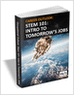MaterialsDesign CenterProcessesUnit ConversionFormulasMathematicsCalculatorsDiscussion ForumTrade PublicationsDirectory Service
STEM Career Outlook

Wages, employment opportunities, and growth projections for STEM jobs.

Salary Expectation

8 things to know about the interview question "What's your salary expectation"?

Learn the best principles to negotiate the salary you deserve!

Injection Molding Design Guide

Guide for high quality and cost-effective plastic injection molding.

more free publicationsGlossary » Units » Power (Heat Flow) » Horsepower (cheval-Vapeur)Horsepower (cheval-Vapeur) (cv, HP) is a unit in the category of Power (Heat flow). It is also known as horsepowers. Horsepower (cheval-Vapeur) (cv, HP) has a dimension of ML2T-3 where M is mass, L is length, and T is time. It can be converted to the corresponding standard SI unit W by multiplying its value by a factor of 735.49875.
Note that the seven base dimensions are M (Mass), L (Length), T (Time), Q (Temperature), N (Aamount of Substance), I (Electric Current), and J (Luminous Intensity).

Other units in the category of Power (Heat flow) include Abwatt (emu of Power) (aW), British Thermal Unit (IT) Per Hour (Btu (IT)/h), British Thermal Unit (IT) Per Minute (Btu (IT)/min), British Thermal Unit (IT) Per Second (Btu (IT)/s), British Thermal Unit (therm.) Per Hour (Btu (therm.)/h), British Thermal Unit (therm.) Per Minute (Btu (therm.)/min), British Thermal Unit (therm.) Per Second (Btu (therm.)/s), Calorie (IT) Per Hour (cal (IT)/h), Calorie (IT) Per Minute (cal (IT)/min), Calorie (IT) Per Second (cal (IT)/s), Calorie (therm.) Per Hour (cal (therm.)/h), Calorie (therm.) Per Minute (cal (therm.)/min), Calorie (therm.) Per Second (cal (therm.)/s), Cheval-Vapeur (horsepower) (cv, HP), Dyne-Centimeter Per Second (dyn-cm/s), Erg Per Second (erg/s), Foot-Pound Force Per Hour (ft-lbf/h), Foot-Pound Force Per Minute (ft-lbf/min), Foot-Pound Force Per Second (ft-lbf/s), Foot-Poundal Per Second (ft-pdl/s), Horsepower (550 Ft-Lbf/s) (HP), Horsepower (boiler) (HP), Horsepower (British) (BHP, hp), Horsepower (electric) (HP), Horsepower (metric) (HP), Horsepower (water) (HP), Kilogram Force-Meter Per Second (kgf-m/s), Kilowatt (kW), Prony, Ton (refrigeration, UK) (CTR (UK)), Ton (refrigeration, US) (CTR (US)), Volt-Ampere (VA), Watt (W), Watt (int. Mean) (W), and Watt (int. US) (W).N/ARelated PagesSTEM Career Outlook

Wages, employment opportunities, and growth projections for STEM jobs.Salary Expectation

8 things to know about the interview question "What's your salary expectation"?